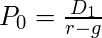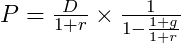Warning: "continue" targeting switch is equivalent to "break". Did you mean to use "continue 2"? in /home/calcul9/public_html/wp-content/themes/suffusion/functions/media.php on line 580

Warning: "continue" targeting switch is equivalent to "break". Did you mean to use "continue 2"? in /home/calcul9/public_html/wp-content/themes/suffusion/functions/media.php on line 583

Warning: "continue" targeting switch is equivalent to "break". Did you mean to use "continue 2"? in /home/calcul9/public_html/wp-content/themes/suffusion/functions/media.php on line 586

Warning: "continue" targeting switch is equivalent to "break". Did you mean to use "continue 2"? in /home/calcul9/public_html/wp-content/themes/suffusion/functions/media.php on line 589

Warning: "continue" targeting switch is equivalent to "break". Did you mean to use "continue 2"? in /home/calcul9/public_html/wp-content/themes/suffusion/functions/media.php on line 592
Fun with the Gordon Growth Model » The Calculating Investor

# Fun with the Gordon Growth Model

May 182011
##### Thinking about Valuation and Mean-Reversion in the Context of a Simple Discounted Cash Flow Model

The Gordon growth model is a simple discounted cash flow (DCF) model which can be used to value a stock, mutual fund, or even the entire stock market.  The model is named after Myron Gordon who first published the model in 1959.

The Gordon model assumes that a financial security pays a periodic dividend (D) which grows at a constant rate (g). These growing dividend payments are assumed to continue forever. The future dividend payments are discounted at the required rate of return (r) to find the price (P) for the stock or fund.

Under these simple assumptions, the price of the security is given by this equation:In this equation, I’ve used the “0” subscript on the price (P) and the “1” subscript on the dividend (D) to indicate that the price is calculated at time zero and the dividend is the expected dividend at the end of period one. However, the equation is commonly written with these subscripts omitted.

Obviously, the assumptions built into this model are overly simplistic for many real-world valuation problems. Many companies pay no dividends, and, for those that do, we may expect changing payout ratios or growth rates as the business matures.

Despite these limitations, I believe spending some time experimenting with the Gordon model can help develop intuition about the relationship between valuation and return.

##### Deriving the Gordon Growth Model Equation

The Gordon growth model calculates the present value of the security by summing an infinite series of discounted dividend payments which follows the pattern shown here:Multiplying both sides of the previous equation by (1+g)/(1+r) gives:We can then subtract the second equation from the first equation to get:Rearranging and simplifying:Finally, we can simplify further to get the Gordon growth model equation:##### Valuation, Discount Rates, and Mean-Reversion

Many investors, myself included, pay close attention to market valuation.  Metrics such as the P/E ratio, Shiller’s PE10 ratio, or the P/D ratio can be used to determine whether the market is relatively expensive or relatively cheap.

Historically, when the market has been relatively cheap, returns over the following 10 to 20 years have tended to be higher than the historical average, and, when the market is relatively expensive, returns over the next 10 to 20 years have tended to be lower than the historical average.  I wrote about using PE10 to forecast returns in a previous market valuation post.

P/D ratios can also be used to forecast market long-term market returns as shown in this WSJ op-ed on market valuation by University of Chicago professor John Cochrane.

By rearranging the terms in the equation, the Gordon growth model can be used to relate the P/D ratio to dividend growth and the discount rate.For a fixed growth rate, g, we can relate P/D to the discount rate r. The graph below shows how P/D relates to r for fixed growth rate of 5%. This 5% matches the nominal rate of growth for S&P500 dividends over the past 50 years.The P/D ratio is clearly sensitive to the discount rate, and this sensitivity increases as the discount rate gets closer to the growth rate. For example, at a discount rate of 9% the P/D ratio is 25. If we lower the discount rate to 8%, the P/D ratio increases to 33, and if we raise the discount rate to 10% the P/D ratio is 20.

This is quite a large valuation range for a reasonable change in discount rate. Discount rates may shift over time as investors change preferences for current vs. future consumption or change attitudes towards risk.

The sensitivity of valuation measures to small changes in the discount rate is the primary reason that I’m skeptical of strong arguments for mean reversion in market valuation. I do believe that some mean reversion is likely in times of extreme market valuation, but I also think that the high sensitivity of valuations to discount rates implies that there is a wide range of valuation levels which could be considered to be “normal”.  The mean level of market valuation over the next 50 years may be very different from the mean value over the past 50 years.

For this reason, I am cautious when I hear statements in the media along the lines of  “stocks are now 30% overvalued“. As the Gordon model shows, a 30% swing in valuation may actually require only a small reduction in the discount rate, and there is no certainty that the discount rate will revert to the historical average value.

##### Conclusion

The Gordon growth model is a useful tool for developing intuition about the relationship between growth rates, discount rates, and valuation.

The simple analysis presented in this post demonstrates that valuations can be quite sensitive to shifts in the discount rate. If you believe that the rate of return that market participants demand may undergo permanent shifts as our economy evolves then you should be somewhat skeptical that market valuations are destined to revert to some historical mean level.

On the other hand, the Gordon growth model does show that, for a given growth rate, there is a clear link between valuation and return. Thus, while it may be very difficult to time the market by analyzing market valuation, it is useful to think about market valuation levels when planning what future levels or return are most likely.

The spreadsheet used to generate the plot showing P/D ratio vs. discount rate can be accessed here.

### 6 Responses to “Fun with the Gordon Growth Model”

1.Thxs for the explanation.

2.Gordon growth model is a simple method for valuing a company. however, its simplicity raises more questions than it answers. Thank you more explaining some of the grey areas of the valuation.

3.thank you

4.double thumps up for explaining the derivation

5.Easy to master thanks

6. […] The basic idea behind DCF analysis is as follows. We start with a basic equation called Gordon’s Growth Model, P = D / (r – g), where P is price, D is dividend, r is the required rate of return, and g is the growth of dividends over time. There’s a very elegant proof of this equation, which you can read about here. […]